# Applied Discrete Structures

## Section9.3Connectivity

This section is devoted to a question that, when posed in relation to the graphs that we have examined, seems trivial. That question is: Given two vertices, $$s$$ and $$t\text{,}$$ of a graph, is there a path from $$s$$ to $$t\text{?}$$ If $$s = t\text{,}$$ this question is interpreted as asking whether there is a circuit of positive length starting at $$s\text{.}$$ Of course, for the graphs we have seen up to now, this question can be answered after a brief examination.

### Subsection9.3.1Preliminaries

There are two situations under which a question of this kind is nontrivial. One is where the graph is very large and an “examination” of the graph could take a considerable amount of time. Anyone who has tried to solve a maze may have run into a similar problem. The second interesting situation is when we want to pose the question to a machine. If only the information on the edges between the vertices is part of the data structure for the graph, how can you put that information together to determine whether two vertices can be connected by a path?

#### Note9.3.1.Connectivity Terminology.

Let $$v$$ and $$w$$ be vertices of a directed graph. Vertex $$v$$ is connected to vertex $$w$$ if there is a path from $$v$$ to $$w\text{.}$$ Two vertices are strongly connected if they are connected in both directions to one another. A graph is connected if, for each pair of distinct vertices, $$v$$ and $$w\text{,}$$ $$v$$ is connected to $$w$$ or $$w$$ is connected to $$v\text{.}$$ A graph is strongly connected if every pair of its vertices is strongly connected. For an undirected graph, in which edges can be used in either direction, the notions of strongly connected and connected are the same.
(Indirect): Suppose $$u$$ is connected to $$w\text{,}$$ but the shortest path from $$u$$ to $$w$$ has length $$m\text{,}$$ where $$m>n\text{.}$$ A vertex list for a path of length $$m$$ will have $$m + 1$$ vertices. This path can be represented as $$\left(v_0,v_1,\ldots, v_m\right)\text{,}$$ where $$v_0=u$$ and $$v_m= w\text{.}$$ Note that since there are only $$n$$ vertices in the graph and $$m$$ vertices are listed in the path after $$v_0\text{,}$$ we can apply the pigeonhole principle and be assured that there must be some duplication in the last $$m$$ vertices of the vertex list, which represents a circuit in the path. This means that our path of minimum length can be reduced, which is a contradiction.

The main advantage of the adjacency matrix method is that the transitive closure matrix can answer all questions about the existence of paths between any vertices. If $$G^+$$ is the matrix of the transitive closure, $$v_i$$ is connected to $$v_j$$ if and only if $$\left(G^+\right)_{i j }=1\text{.}$$ A directed graph is connected if $$\left(G^+\right)_{i j }=1$$ or $$\left(G^+\right)_{j i}=1$$ for each $$i\neq j\text{.}$$ A directed graph is strongly connected if its transitive closure matrix has no zeros.
A disadvantage of the adjacency matrix method is that the transitive closure matrix tells us whether a path exists, but not what the path is. The next algorithm will solve this problem.

### Subsection9.3.4Graph Measurements

If we were to perform a breadth first search from each vertex in a graph, we could proceed to determine several key measurements relating to the general connectivity of that graph. From each vertex $$v\text{,}$$ the distance from $$v$$ to any other vertex $$w\text{,}$$ $$d(v,w)\text{,}$$ is number of edges in the shortest path from $$v$$ to $$w\text{.}$$ This number is also the index of the depth set to which $$w$$ belongs in a breath-first search starting at $$v\text{.}$$
\begin{equation*} d(v,w) = i \iff w \in D_v(i) \end{equation*}
where $$D_v$$ is the family of depth sets starting at $$v\text{.}$$
If the vector of “from-values” is known from the breath-first search, then the distance can be determined recursively as follows:
\begin{equation*} d(v,v) =0 \end{equation*}
\begin{equation*} d(v,w) = 1 + d(v,w.from) \textrm{ if }w\neq v \end{equation*}
Consider Figure 9.3.13. If we perform a breadth first search of this graph starting at vertex 2, for example, we get the following “from data” telling us from what vertex each vertex is reached.
\begin{equation*} \begin{array}{ccccccccccccc} \text{vertex} & 1 & 2 & 3 & 4 & 5 & 6 & 7 & 8 & 9 & 10 & 11 & 12 \\ \text{vertex.from} & 7 & 2 & 10 & 6 & 9 & 7 & 2 & 4 & 2 & 7 & 9 & 2 \\ \end{array} \end{equation*}
For example, 4.from has a value of 6. We can compute $$d(2,4)\text{:}$$
\begin{equation*} \begin{split} d(2,4) &= 1+d(2,4.from)= 1+d(2,6)\\ &=2+d(2,6.from)=2+d(2,7)\\ &=3+d(2,7.from)=3+d(2,2)\\ &=3 \end{split} \end{equation*}
Once we know distances between any two vertices, we can determine the eccentricity of each vertex; and the graph’s diameter, radius and center. First, we define these terms precisely.
Eccentricity of a Vertex
The maximum distance from a vertex to all other vertices, $$e(v)=\max_{w}\,d(v,w)\text{.}$$
Diameter of a Graph
The maximum eccentricity of vertices in a graph, denoted $$d(G)\text{.}$$
The minimum eccentricity of vertices in a graph, denoted $$r(G)\text{.}$$
Center of a Graph
The set of vertices with minimal eccentricity, $$C(G)=\{v\in V \mid e(v)=r(G)\}$$
If we compute all distances between vertices, we can summarize the results in a distance matrix, where the entry in row $$i\text{,}$$ column $$j$$ is the distance from vertex $$i$$ to vertex $$j\text{.}$$ For the graph in Example 9.3.12, that matrix is
\begin{equation*} \left( \begin{array}{cccccccccccc} 0 & 2 & 2 & 2 & 3 & 1 & 1 & 3 & 3 & 1 & 2 & 2 \\ 2 & 0 & 3 & 3 & 2 & 2 & 1 & 4 & 1 & 2 & 2 & 1 \\ 2 & 3 & 0 & 2 & 5 & 3 & 2 & 3 & 4 & 1 & 4 & 3 \\ 2 & 3 & 2 & 0 & 3 & 1 & 2 & 1 & 3 & 1 & 2 & 3 \\ 3 & 2 & 5 & 3 & 0 & 2 & 3 & 4 & 1 & 4 & 1 & 3 \\ 1 & 2 & 3 & 1 & 2 & 0 & 1 & 2 & 2 & 2 & 1 & 2 \\ 1 & 1 & 2 & 2 & 3 & 1 & 0 & 3 & 2 & 1 & 2 & 1 \\ 3 & 4 & 3 & 1 & 4 & 2 & 3 & 0 & 4 & 2 & 3 & 4 \\ 3 & 1 & 4 & 3 & 1 & 2 & 2 & 4 & 0 & 3 & 1 & 2 \\ 1 & 2 & 1 & 1 & 4 & 2 & 1 & 2 & 3 & 0 & 3 & 2 \\ 2 & 2 & 4 & 2 & 1 & 1 & 2 & 3 & 1 & 3 & 0 & 3 \\ 2 & 1 & 3 & 3 & 3 & 2 & 1 & 4 & 2 & 2 & 3 & 0 \\ \end{array} \right) \end{equation*}
If we scan the matrix, we can see that the maximum distance is the distance between vertices 3 and 5, which is 5 and is the diameter of the graph. If we focus on individual rows and identify the maximum values, which are the eccentricities, their minimum is 3, which the graph’s radius. This eccentricity value is attained by vertices in the set $$\{1, 4, 6, 7\}\text{,}$$ which is the center of the graph.

### Exercises9.3.6Exercises

#### 1.

Apply Algorithm 9.3.8 to find a path from 5 to 1 in Figure 9.3.11. What would be the final value of $$V\text{?}$$ Assume that the terminal vertices in edge lists and elements of the depth sets are put into ascending order, as we assumed in Example 9.3.10.
$$\begin{array}{ccccccc} k & 1 & 2 & 3 & 4 & 5 & 6 \\ V[k].\text{found} & T & T & T & F & F & T \\ V[k].\text{from} & 2 & 5 & 6 & * & * & 5 \\ \text{Depth} \text{Set} & 2 & 1 & 2 & * & * & 1 \\ \end{array}$$ $$\text{(*} = \text{undefined})$$

#### 2.

Apply Algorithm 9.3.8 to find a path from $$d$$ to $$c$$ in the road graph in Example 9.1.7 using the edge list in that example. Assume that the elements of the depth sets are put into ascending order.

#### 3.

In a simple undirected graph with no self-loops, what is the maximum number of edges you can have, keeping the graph unconnected? What is the minimum number of edges that will assure that the graph is connected?
If the number of vertices is $$n\text{,}$$ there can be $$\frac{(n-1)(n-2)}{2}$$ vertices with one vertex not connected to any of the others. One more edge and connectivity is assured.

#### 4.

Use a broadcasting algorithm to determine the shortest path from vertex $$a$$ to vertex $$i$$ in the graphs shown in the Figure 9.3.15 below. List the depth sets and the stack that is created.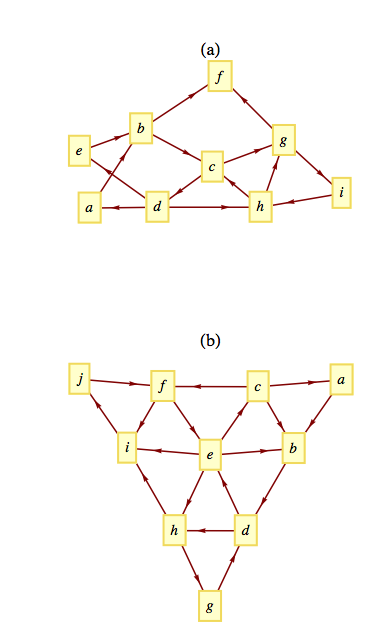Figure 9.3.15. Shortest paths from $$a$$ to $$i\text{?}$$

#### 5.

For each of the following graphs, determine the eccentricities of each vertex, and the diameter, radius, and center of the graph.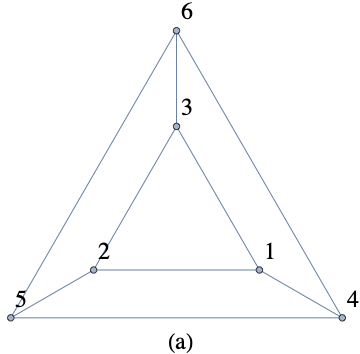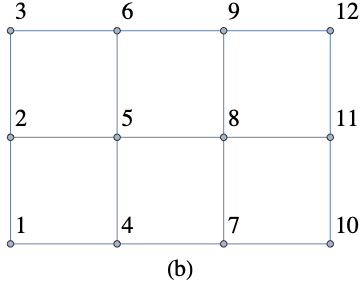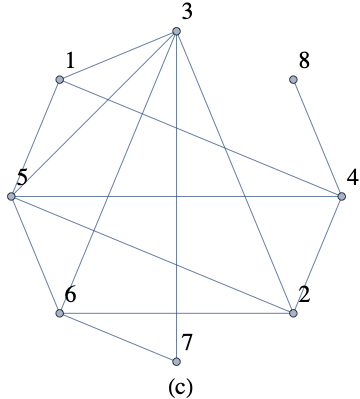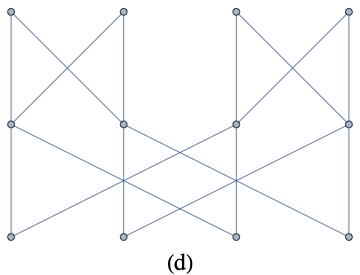1. The eccentricity of each vertex is 2; and the diameter and radius are both 2 as well. All vertices are part of the center.
2. The corners (1,3,10 and 10) have eccentricities 5. The two central vertices, 5 and 8, which are in the center of the graph have eccentricity 3. All other vertices have eccentricity 4. The diameter is 5. The radius is 3.
3. Vertices 1, 2 and 5 have eccentricity 2 and make up the center of this graph. Verticies 7 and 8 have eccentricity 4, and all other vertices have eccentricity 3. The diameter is 4. The radius is 2.
4. The eccentricity of each vertex is 4; and the diameter and radius are both 4 as well. All vertices are part of the center.

#### 6.

1. The terms diameter, radius and center are familiar ones in the context of circles. Compare their usage in circles and graphs. How are they similar and how are they different?
2. “Eccentricity” might be less familiar. How is is used in geometry, and does it have a compatible use in graph theory?

#### 7.

Prove (by induction on $$k$$) that if the relation $$r$$ on vertices of a graph is defined by $$v r w$$ if there is an edge connecting $$v$$ to $$w\text{,}$$ then $$r^k\text{,}$$ $$k \geq 1\text{,}$$ is defined by $$v r^kw$$ if there is a path of length $$k$$ from $$v$$ to $$w\text{.}$$
Basis: $$(k=1)$$ Is the relation $$r^1\text{,}$$ defined by $$v r^1 w$$ if there is a path of length 1 from $$v \text{ to } w\text{?}$$ Yes, since $$v r w$$ if and only if an edge, which is a path of length $$1\text{,}$$ connects $$v$$ to $$w\text{.}$$
Induction: Assume that $$v r^k w$$ if and only if there is a path of length $$k$$ from $$v$$ to $$w\text{.}$$ We must show that $$v r^{k+1} w$$ if and only if there is a path of length $$k+1$$ from $$v$$ to $$w\text{.}$$
By the induction hypothesis, there is a path of length $$k$$ from $$v \textrm{ to } y\text{.}$$ And by the basis, there is a path of length one from $$y$$ to $$w\text{.}$$ If we combine these two paths, we obtain a path of length $$k+1$$ from $$v$$ to $$w\text{.}$$ Of course, if we start with a path of length $$k+1$$ from $$v$$ to $$w\text{,}$$ we have a path of length $$k$$ from $$v$$ to some vertex $$y$$ and a path of length 1 from $$y$$ to $$w\text{.}$$ Therefore, $$v r^k y \textrm{ and } y r w \Rightarrow v r^{k+1} w\text{.}$$
For each of the following distance matrices of graphs, identify the diameter, radius and center. Assume the graphs vertices are the numbers 1 through $$n$$ for an $$n \times n$$ matrix.
1. $$\displaystyle \left( \begin{array}{cccccccccc} 0 & 2 & 1 & 2 & 2 & 3 & 3 & 2 & 1 & 1 \\ 2 & 0 & 1 & 2 & 3 & 3 & 3 & 2 & 3 & 2 \\ 1 & 1 & 0 & 1 & 2 & 2 & 2 & 1 & 2 & 1 \\ 2 & 2 & 1 & 0 & 3 & 3 & 3 & 2 & 3 & 2 \\ 2 & 3 & 2 & 3 & 0 & 2 & 1 & 1 & 2 & 1 \\ 3 & 3 & 2 & 3 & 2 & 0 & 1 & 1 & 3 & 2 \\ 3 & 3 & 2 & 3 & 1 & 1 & 0 & 1 & 3 & 2 \\ 2 & 2 & 1 & 2 & 1 & 1 & 1 & 0 & 2 & 1 \\ 1 & 3 & 2 & 3 & 2 & 3 & 3 & 2 & 0 & 1 \\ 1 & 2 & 1 & 2 & 1 & 2 & 2 & 1 & 1 & 0 \\ \end{array} \right)$$
2. $$\displaystyle \left( \begin{array}{cccccccccccc} 0 & 2 & 2 & 2 & 3 & 3 & 3 & 1 & 2 & 3 & 1 & 1 \\ 2 & 0 & 2 & 2 & 1 & 1 & 1 & 3 & 2 & 1 & 1 & 3 \\ 2 & 2 & 0 & 1 & 3 & 2 & 1 & 2 & 2 & 3 & 1 & 1 \\ 2 & 2 & 1 & 0 & 3 & 1 & 2 & 1 & 2 & 3 & 2 & 1 \\ 3 & 1 & 3 & 3 & 0 & 2 & 2 & 4 & 3 & 2 & 2 & 4 \\ 3 & 1 & 2 & 1 & 2 & 0 & 2 & 2 & 3 & 2 & 2 & 2 \\ 3 & 1 & 1 & 2 & 2 & 2 & 0 & 3 & 3 & 2 & 2 & 2 \\ 1 & 3 & 2 & 1 & 4 & 2 & 3 & 0 & 3 & 4 & 2 & 2 \\ 2 & 2 & 2 & 2 & 3 & 3 & 3 & 3 & 0 & 1 & 3 & 1 \\ 3 & 1 & 3 & 3 & 2 & 2 & 2 & 4 & 1 & 0 & 2 & 2 \\ 1 & 1 & 1 & 2 & 2 & 2 & 2 & 2 & 3 & 2 & 0 & 2 \\ 1 & 3 & 1 & 1 & 4 & 2 & 2 & 2 & 1 & 2 & 2 & 0 \\ \end{array} \right)$$